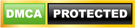Blog

# TensorFlow Tutorial

• ###### | 1794 Ratings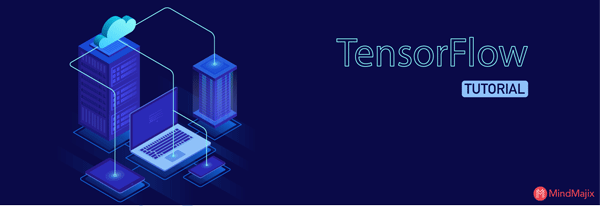Deep learning is a part of machine learning methods where neural networks, algorithms, inspired by human brain learn from large amount of datasets. Similarly how a human being learn from his past experiences, deep learning algorithms will perform some task repeatedly to produce an improved outcome in each run. The more deep learning algorithms learn, the better they perform.It can be considered to automate the predictive analytics.

In this article, we will be covering below topics to gain in-depth knowledge on TensorFlow.

### What is TensorFlow?

TensorFlow is an open source machine learning library, invented by Google, and used to design, construct, and train deep learning models. TensorFlow is a library for dataflow programming. It has numerous optimization techniques to make mathematical expressions’ complexity easier and more performant.

#### Below are some key features of TensorFlow:

• It works efficiently with mathematical expressions of multi-dimensional arrays.
• It has GPU/CPU computing due to which we can execute the same code on both architectures.
• It has great support of machine learning concepts and deep neural networks
• It is highly scalable for computations across machines and large datasets.
• Altogether, these key features make TensorFlow shine as a perfect framework for machine intelligence.

### What are Tensors?

Before understanding TensorFlow and how it works, let us first understand what actually a Tensor is.
A tensor is a mathematical representation of a physical entity which can be described in multiple directions or magnitude. Tensors are multidimensional arrays of base data types. Each element in Tensor is of the same datatype.  Tensor consists of 2 main properties: Data Type and Shape. Tensor’s data type is always known whereas its shape can be known partially. Tensor shape is defined as a number of dimensions it has and size of each dimension.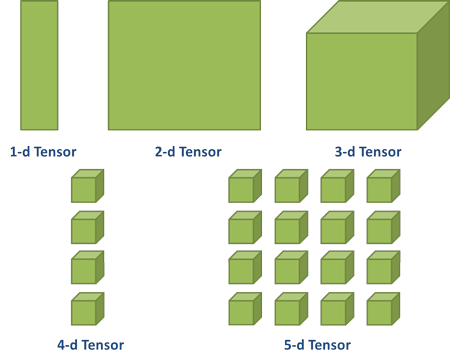While writing TensorFlow code, you usually work with a Tensor object called tf.Tensor. This object is a representation of a partially defined computation, which will produce a value at the end. Tensor is identified by its rank. Rank of Tensor is nothing but its number of dimensions. Tensor is described using 3 notational conventions – rank, shape and dimension number.  Each rank here is attached to a different mathematical entity as described below:

 Rank Math Entity Shape Dimension Example 0 Scalar (magnitude only) [] 0-D A 0-D tensor. A scalar. 1 Vector (magnitude and direction) [D0] 1-D A 1-D tensor with shape . 2 Matrix (table of numbers) [D0,D1] 2-D A 2-D tensor with shape [3, 4]. 3 3-Tensor (cube of numbers) [D0,D1,D2] 3-D A 3-D tensor with shape [1, 4, 3]. N n-Tensor [D0,D1,D2,…..,Dn-1) n-D A tensor with shape [D0, D1, ... Dn-1].

### Installing TensorFlow

Now, as we are aware of what TensorFlow is, let’s first install the library to kick start learning.
Download a version of TensorFlow which enables us to write the code for deep learning projects in Python. This is easily available on TensorFlow’s website. On this website, multiple ways are available to install TensorFlow such as using pip, Docker, etc.

pip install tensorflow

Once we follow the instructions given on the official website, let us verify whether TensorFlow installed correctly or not by importing it into our workspace using below command:

import tensorflow as tf

TensorFlow provides API for various programming languages like Python, Java, Go, Rust, Haskell, C++ and R.

### Dataflow Graph in TensorFlow

TensorFlow is made up of Tensor and Flow. Tensor is a representation of data into multidimensional arrays. Flow is defined as sequences of operations performed on these Tensors.

Let us explore what data flow graphs are. Computations are represented as data flow graphs. Edges are Tensors flowing through the graph, (multidimensional arrays) whereas nodes are operations.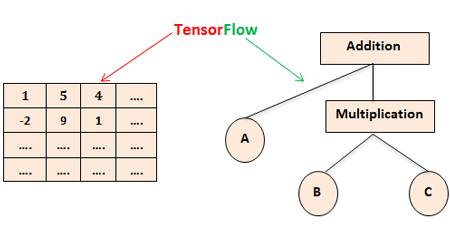Edges in TensorFlow can be categorized into 2 groups:

1. Normal Edges: They transfer tensors and output of one operation can become input for another.
2. Special Edges: They controls the dependencies between two nodes and set the preference and wait for one to finish

### TensorFlow Code Basics:

Constants, Variables, Sessions and Placeholders

#### Constants

Constants are created using below signature in TensorFlow

constant(value, dtype = None, shape = None,  name = ‘cost’, verify_shape = false)

Here, value is an actual constant value which will be used in computation. Constants are taking 0 inputs and producing constant stored value as output. Below here is an example for the same:

b = tf.constant(5)

#### Variables

In TensorFlow, variables are considered as an in-memory buffer which contains tensors in it. These variables need to be initialized explicitly and are used to maintain state in graph. Variables can be defined as mentioned below:

v = tf.Variable(, dtype = tf.float32)

Here the data type is optional. If we don’t define any variable’s data type, TensorFlow will decide the type of variable from the initialized value.

We need to initialize variables explicitly before using it in graph. Below are the commands to initialize variables before using it in graph for the first time:

init = tf.global_variables_initializer()
sess.run(init)

#### Placeholders

Placeholder is same like a constant or a variable which stores value in it. But the only difference it possesses is the value will be provided to placeholder at runtime. The syntax for placeholder is mentioned below:

placeholder(dtype, shape=None, name=None)

A placeholder tensor must feed with data while runtime else flow will generate an error. Placeholders make the computation graph generic in nature. We can run the same code with different values multiple times without re-writing same code again and again for different values.

#### Sessions

Any computation graph running in a session will evaluate actual values of nodes. A session is defined as the state and control of TensorFlow runtime. It stores in which order all operations will be performed and pass the result of one node to next node in the pipeline.

### How to build and run a Computational Graph?

TensorFlow program architecture is mainly divided into 2 steps:

#### Build a Computational Graph

A computational graph is a directed graph where nodes are variables and edges are operations on those variables. Operations are fed with variable values and they can transfer their output to other operations as well. These values which fed into nodes and come out of nodes are called Tensors.

Let us understand more about computational graph through below example:

Consider a function C: R2 → R where c(a,b) = a+b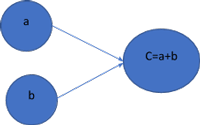This computational graph computes the sum of input variables a and b and store it into c. Computational graphs provide an alternative way to perform mathematical calculations. The operations assigned to different nodes can be performed in parallel which improves performance in computations.

Code template for above computation graph is as below:

```import tensorflow as tf
a = tf.constant(3.0)
b = tf.constant(4.0)
c = a + b```

This concept shows its usefulness when computations become more complex.

For example: linear regression model expression c(A,x,b) = Ax + b. We will see how we create linear regression computation graph further in this article.

#### Run a Computational Graph

To run a computational graph, we need a session to execute operations. Session provides control and state of TensorFlow runtime. Session contains the sequence of operations and passes the result of one computation to another.

Below is the code which shows how can we run above sum example into a session and compute the output:

```session = tf.Session()
output = session.run(c)
print(output)
```
```Output:
7```

This is how a computation graph works in a session. While building the graph, we need to make sure the operations are in a correct order.

### Linear Regression Model with TensorFlow

Linear Regression Model is used to evaluate the dependent variable from the other known variables using linear regression equation. Computation graph for Linear expression c(A,x,b) = Ax + b is as below: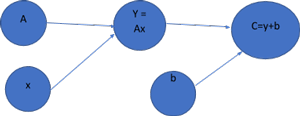For this, we need 4 variables

• Output variable C
• Input Variable x
• Slope variable A
• Y-Intercept or bias - b

Now, let’s implement code for this TensorFlow.

```# Creating slope variable with initial value as 0.5
A = tf.Variable([.5], tf.float32)

#Creating bias variable b for with initial value as -0.5
b = tf.Variable([-0.5], tf.float32)

# Creating placeholders for providing input or independent variable x
x = tf.placeholder(tf.float32)

# Equation of Linear Regression
linear_exp = A * x + b

# Initializing all the variables with below commands
s = tf.Session()
i = tf.global_variables_initializer()
s.run(i)

# Running linear regression model to compute the output for provided x values
print(s.run(linear_exp {x: [1,2,3]}))

```
```Output:
[0 0.5 1]```

While building a regression model, we need to consider below 2 things:

• How far is our computed output with desired or targeted output?
• We need to give a proper mechanism through which our model train itself based on given inputs and respective outputs.

#### Model Validation

For model validation, we are using Loss function which compares our training model outputs with desired or targeted outputs. There is a most common loss function for linear regression model called S.S.E. (Sum of Squared Error).

E = ½*(t-y)2
E: Mean Squared Error
t: targeted output
y: actual output
(t-y): error

If we are getting high loss value, then we need to adjust our scalar variable bias and weight variable to decrease the error we are getting.

#### Training the Model

To optimize the loss function, TensorFlow provides optimizers which change variables slowly to minimize the loss value. Gradient descent is the simplest of this kind. It modifies variables according to the loss function’s magnitude with respect to the variable. Below is the basic algorithm of how this works: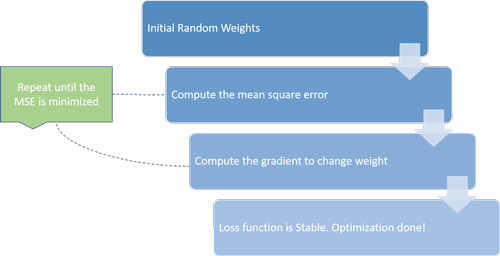Gradient descent will iterate the process until the error is reduced. Each process is termed as an iteration. If learning rate is too small, then algorithms will take a long time to converge as it requires lot of iterations. If learning rate is too high then algorithms might never converge.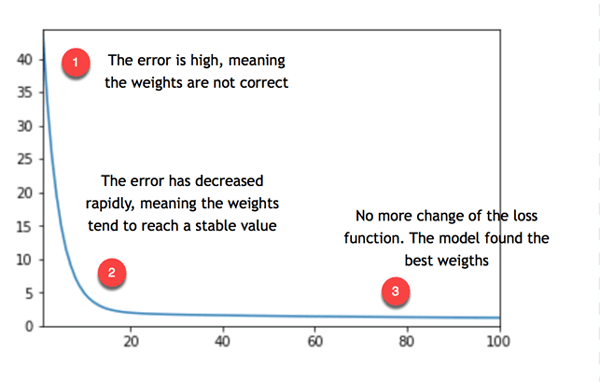Model is repeating their process until its weights reach to a stable value. If error is not 0 and it stabilized around different number like 5, as shown in this picture, that means the model is making a typical error as 5. To reduce it, we need to add some more information to model, like more variables or estimators.

So, this is how we can build a linear regression model and train it for desired output.

### Conclusion

TensorFlow is a powerful framework which makes working with mathematical expressions and multi-dimensional arrays easier which is the most important aspect of machine learning. It also reduces the complexities of scaling and executing data graphs.

In the course of time, TensorFlow has become popular and now majorly used by developers for solving problems using deep learning methods for video detection, image recognition, text processing like sentiment analysis etc. TensorFlow has great documentation and community support. If we follow that, it will become a less tedious process to solve the problems with TensorFlow. TensorFlow is used by all major Tech giants like Dropbox, Snapchat, twitter, Airbnb, eBay, SAP, IBM, Uber, Qualcomm for image recognition. Moreover, Facebook, Google, Instagram and Amazon also use TensorFlow for different purposes. Most of all tech companies are using it in one or another way. This can be the biggest reason to learn and know more about TensorFlow.

Now that you know about the importance of Deep Learning and TensorFlow, check out Mindmajix course to learn TensorFlow in detail and crack the interview at your dream company.